# CS 570, Analysis of Algorithms, Spring 2012

 Time and Location MW, 8:30–9:50am, ZHS 352 Instructor Prof. Liang Huang (liangh@usc) Teaching Assistant Kai Song (kaisong@usc) Grader Phani Chaitanya Vempaty (vempaty@usc) Course Homepage http://www.isi.edu/~lhuang/teaching/cs570/ Office Hours LH: MW, 10–11am, SAL 234 KS: T 9–11am, SAL 235 Additional office hours available before midterms and final. Textbooks [CLRS] Introduction to Algorithms, 3rd or 2nd edi. (default reference). (assignments refer to the 3rd edition). [KT] Kleinberg and Tardos, Algorithm Design (also recommended) Grading homework: 2%x6=12%, quizzes: 6%x3=18%, midterms: 15%+25%=40%, final: 30%. homework policy: discussions are fine, but each student must writes up his/her own solutions.

 type programming analysis of algorithms (time/space complexities, worst/best-case scenarios, counterexamples) algorithm design proof of correctness homework yes yes yes yes quizzes no yes occasionally no exams no yes yes occasionally

Topics Covered
• Introduction: Some Interesting Problems
• Runtime Analysis and Big-O Notation: Master Theorem
• Divide and Conquer, Sorting and Selection: Quicksort, Quickselect, and Mergesort
• Data Structures: Heaps and Heapsort, Hash Tables, and Binary Search Trees
• Dynamic Programming
• Graph Algorithms I: DFS, BFS, Topological Sort, Strongly Connected Components
• Graph Algorithms II: Shortest Paths (Dijkstra) and Minimum Spanning Tree (Kruskal and Prim)
• Graph Algorithms III: Network Flow (Ford-Fulkerson)
• Computational Geometry: Convex Hulls (if time permits)
• NP-Completeness
Syllabus

WeekDateTopics and Readings (CLRS and KT)HW/Quiz/Exams
1 Mon 1/9
• Intro: longest increasing subsequence
• greedy: wrong. O(n)
• brute force: correct. O(2^n). powerset construction
• Big-O informal intro
• quicksort example
-
Wed 1/11
• longest increasing subsequence (wikipedia)
• dynamic programming: correct, O(n^2).
• backtracing to print the solution.
• proof of correctness by (complete) induction
• it's possible for O(nlogn) with binary search (beyond this course)
• insertion sort (CLRS 2.3)
• proof of correctness by (simple) induction
• improvements: binary search or linkedlist, but not both
• complexity remains O(n^2) anyways
• review of arrays vs. linkedlists (KT 2.3)  - randomaccess insertion/deletion find array O(1) O(n) normal: O(n)sorted: O(logn) hashed: ~O(1) linkedlist O(n) O(1) O(n)
• quick sort (CLRS 7.1-2)
• worst-case scenario
• best-case scenario
• connection to binary search trees (CLRS 12.1-2) but not binary search (CLRS 2.3, KT 2.3)
HW1 out (due Mon 1/23).
2Mon 1/16Martin-Luther King's Day. no class.-
Wed 1/18
• Quiz 1 (20 min.)
• quicksort analysis (CLRS 7.3-4, KT 13.5)
• randomization: shuffling and random pivot
• intuitions why worst-case is rare after randomization
• average-case analysis (expected runtime for randomized quicksort)
(high-level intuitions are important, but details of this proof are not required)
QUIZ 1
3Mon 1/23
• discussions of Quiz 1
• mergesort (CLRS 2.3, KT 5.1)
• merging two sorted list is linear
• substitution method for analyzing complexity
• quicksort vs. mergesort
• why/when quicksort is generally faster? (in place, in memory)
• when is mergesort useful? (linkedlist, files on disk)
• stable sort: mergesort and insertion sort are stable (with careful implemenations)
• quicksort unstable with in-place implementation with randomized pivot
• why stability matters: sorting with multiple keys (last name, first name)
• priority queue / heapsort (CLRS 6)
• complete binary tree, linear (array) representationHW1 due
Wed 1/25
• Big-O, Big-Theta, Big-Omega: formal intro (CLRS 3, KT 2.2).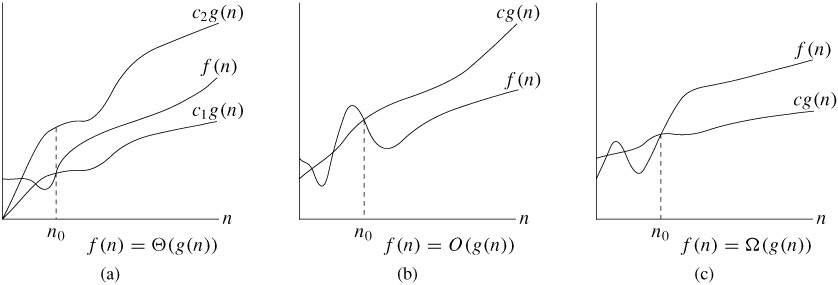• Substitution and Recursion Tree Methods (brief, CLRS 4.3-4)
• Master theorem (CLRS 4.5), examples:
• T(n) = 2T(n/2) + O(n)    (mergesort)
• T(n) = 2T(n/2) + O(1)    (binary tree traversal, cf. quiz 1)
• T(n) = T(n/2) + O(1)      (binary search)
• T(n) = T(n/2) + O(n)      (quickselect)
• heapsort (cont'd)
• priority queue vs. queue: emergency room vs. checkout line
• heap operation: push/insert (add at the end; bubble-up); O(log n)
• heap operation: pop/extract-min (pop root; move the last element to root; bubble-down); O(log n)
• bubble-up and bubble-down are the building-blocks for other heap operations.
HW 2 out
4Mon 1/30
• heapsort (cont'd)
• use priority queue to model stack and queue (CLRS problem 6.5-7, trivial)
• heap operation: change-key (bubble-up or bubble-down)
• heap operation: build heap (from array)
• method 0: sort it first (overkill!): O(nlogn)
• method 1: insert each element: O(nlogn) (CLRS problem 6-1)
• method 2: heapify (bottom-up or recursive). tight analysis: O(n). (CLRS 6.3)
• formal analyses of methods 1 and 2:
useful fact 1: # of elements with height h is n/2^{h+1}.
useful fact 2: 1/2 + 2/4 + 3/8 + ... = (1/2 + 1/4 + 1/8 + ...) + (0 + 1/4 + 2/8 + 3/16 + ...)
= 1 + (1/4 + 1/8 + 1/16 + ...) + (1/8 + 2/16 + 3/32 + ...) = 1 + 1/2 + ... = 2.
(this derivation of fact 2 is more accessible than the one in the textbook).
• method 2: sum O(h) n/(2^{h+1}) = O(n) sum h/2^h = O(n x 2) = O(n).
• method 1: sum O(logn - h) n/(2^{h+1}) = O(nlogn) x sum 1/2^h - O(n) sum h/2^h = O(nlogn) - O(n)=O(nlogn).
• high-level intuitions: method 2 is faster because the majority (lowest levels) requires very little work (bubble-down to the leaves), while method 1 is slow because the majority requires the most work (bubble-up to the root).
• heapsort is O(nlogn): build heap O(n), pop each element O(nlogn).
• example application: k-way mergesort: merging becomes O(k+nlogk)=O(nlogk). (CLRS problem 6.5-9)
Wed 2/1
• review of heapify:
• recursive version: heapify left, heapify right, bubble-down.
T(n)=2T(n/2) + O(logn).
use Master Theorem case 1 (when f(n) small): T(n)=O(n).
this analysis is more intuitive than the sum version in the textbook.
• (where as "keep pushing" is T(n)=T(n-1)+O(logn)=O(nlogn).)
• applications: select kth-smallest element: O(n+klogn). fast when k << n.
• quickselect (CLRS 9.2, KT 13.5)
• idea from quicksort: partition, but throw half away
• best-case O(n): T(n)=T(n/2) + O(n).
use Master Theorem case 3 (check regularlity!), or geometric series.
• worst-case O(n^2): T(n)=T(n-1)+O(n).
• randomized version: expected linear time
• determinstic worst-case linear-time select (CLRS 9.3)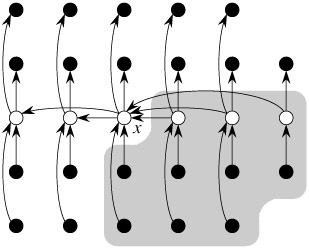• idea: find a balanced pivot to partition w/o randomization
• 5 steps in each recursive call:
• divide into n/5 groups of 5: O(n)
• insertion-sort each group: O(n)
• recursively find x=median-of-medians: T(n/5).
• partition using x: O(n). at least 3n/10 < x, and at least 3n/10 > x.
• recursion on one half: T(7n/10).
• total: T(n)=T(n/5)+T(7n/10)+O(n).
use substitution method, guess T(n)=O(n), i.e., T(n)<=cn for some c. work out the math. T(n)=O(n).
• Why magic number of 5? what about 3, 4, 6, 7? (CLRS problem 9.3-1).
5 is the minimum magic number. e.g., why 4 is not enough:
T(n) = T(n/4) + T(3n/4) + O(n) = O(n^2).
• this algorithm is mostly of theoretical interest (constant overhead too large).
5Mon 2/6
• review of worst-case linear selection
• example applications of selection
• worst-case O(nlogn)-time quicksort based on selection (CLRS problem 9.3-3)
• find k elements closest to median in O(n) time. (CLRS problem 9.3-7)
• find median of two sorted lists in O(logn) time. (CLRS problem 9.3-8)

• lower-bounds for sorting (CLRS 8.1)
decision tree model: each non-leaf node is one comparison, and each leaf node is a complete ordering. the tree should have at least n! leaves: 2^h >= n!, so h >= log n!.
(n/2)^(n/2) <= n! <= n^n, so log n! = \Theta(nlogn). so h = \Omega(nlogn).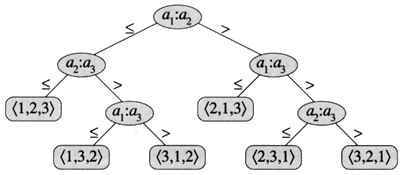HW2 due
Wed 2/8 Topics Covered So Far
• Analysis Notions: Big-{O, Theta, Omega}, Worst-Case, Best-Case, Average-Case
• Analysis Techniques: Master, Substitution, Recursion Tree
• Data Structures: BST, Heap (Priority Queue), LinkedList
• Algorithms
• Sorting: Insertion, Quicksort, Mergesort, Heapsort
• Selection: Quickselect, Worst-case linear select
• Lower-Bounds: Comparison Sort: O(nlogn)
Review Problems
• heapsort is not stable. example: [3a, 3b, 3c]. pop out: 3a, 3c, 3b.
• O(nlogn)-time to check if there exist x+y=S.
• O(logn)-time to find the number in a (balanced) BST that is closest to query x.
• find the k smallest numbers in a data-stream of n numbers with only O(k) space.
• k-way mergesort. Merging is O(k+nlogk)=O(nlogk).
Overall: T(n)=kT(n/k) + O(n log k). Can't use Master Theorem (why?).
• Pitfalls of using substitution method: must prove the exact form. (CLRS 4.3)
Extra office hour Fri 9:30-11:30, SAL 235.
6Mon 2/13 MIDTERM 1 (covers all lectures so far). No office hours on Mon/Tue.
Wed 2/15 Discussions of Midterm 1 problems.regrade session in office hour.
7Mon 2/20PRESIDENT'S DAY -- NO CLASS
Wed 2/22 Dynamic Programming
• Example Problems
• Review of Longest Increasing Subsequence
• The Unbounded Knapsack Problem (see wikipedia)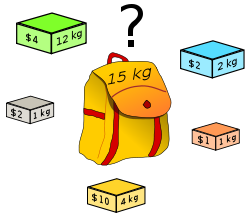• Steps
• Define subproblem
• Recurrence relation
• Reconstructing Optimal Solution
• Implementations
• Bottom-Up
• Top-Down recursive + memoization (e.g. Fibonacci)
• Requirements
• Optimal Substructure (check your subproblem definition)
• Sharing of Subproblems (cf. memoization)
8Mon 2/27 Dynamic Programming (cont'd)
• The 0-1 Knapsack Problem (see wikipedia)

opt[w][i] -- optimal value of a bag of weight w, using items 1..i

• Longest Common Subsequence (Sequence Alignment)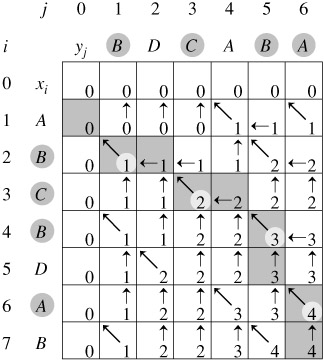opt[i][j] -- LCS b/w A_{1..i} and B_{1..j}
opt[i][j] = max { opt[i][j-1], opt[i-1][j],
opt[i-1][j-1]+1(A_i==B_j) }

applications: sequence alignment (e.g. DNA), edit distance, spelling correction, etc.

• Matrix-Chain Multiplication
Wed 2/29 Dynamic Programming (cont'd)
• Matrix-Chain Multiplications

basics: multiplying a p x q matrix with a q x r matrix results in an p x r matrix and takes p x q x r multiplications.

matrix-chain A_1 A_2 ... A_n.
each A_k has dimensions p_{k-1} x p_k (neighboring pairs share one dimension).

example: A x B x CA x (B x C) is better (2x3x3+3x3x2).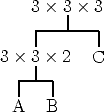vs.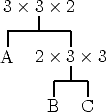objective: find the order of multiplications that minimizes the total # of scalar multiplications.

m[i, j] -- optimal # of multiplications for subchain A_i x ... x A_j.
m[i, j] = min_{i<=k<j} m[i, k] + m[k+1, j] + p_{i-1} p_k p_j
m[i, i] = 0.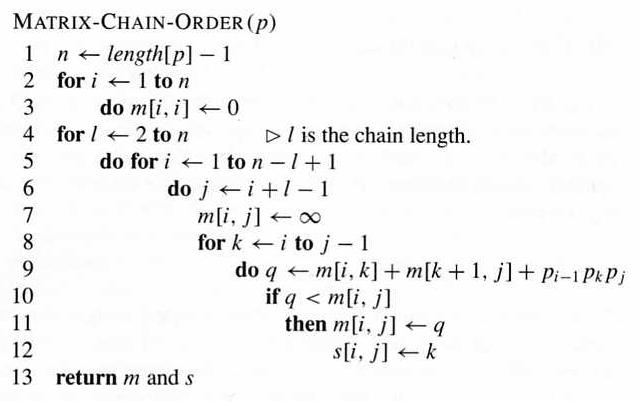complexity: O(n^3) time, O(n^2) space.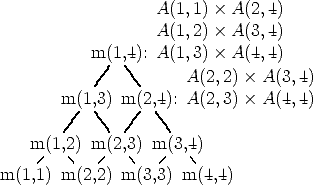fill in the chart. e.g. A1 : 3 x 2, A2 : 2 x 4, A3 : 4 x 3, A4 : 3 x 2

9Mon 3/5 Quiz 2 and discussions; DP on graphs and hypergraphs (matrix-chain)
Wed 3/7 Viterbi algorithm on DAG; topological sort
10SPRING BREAK - NO CLASS
11Mon 3/19
• review on topological sort
• pseudocode: BFS-style
• theorem: the following three are equivalent for directed graph G
• G is acyclic
• G has a valid topological ordering
• the BFS-style topological sort succeeds
• BFS
• connected components for undirected graphs
• strongly-connected components (SCCs) for directed graphs
Wed 3/21 tree traversal review:
[DFS] pre-order, post-order, and (for binary trees only) in-order,
[BFS] level-order.

DFS on directed graphs;
DFS edge classification: tree, back, forward, cross.
DFS for undirected graphs: tree and back edges only.

12Mon 3/26 DFS time intervals (easier to understand than edge classification);
DFS for SCCs: Kosaraju's Algorithm (two DFS's, CLRS 22.5);
SCC-DAG
Wed 3/28 DFS for SCCs: Tarjan's Algorithm (single DFS, see wikipedia).
All topological orders for Matrix-Chain Multiplication DP.
Viterbi algorithm for shortest, longest, and # of paths on DAG.
13Mon 4/2 MIDTERM 2
Wed 4/4 discussions of midterm 2; discussion of HW4; regrading session.

Homework Assignments (due at the beginning of the class on paper only; please print your code)
 out due programming (please print your code!) theory (CLRS, 3rd edi) Solutions HW1 Wed 1/11 Mon 1/23 longest increasing subsequence (both brute force and DP) quicksort; identify worst-case and best-case scenarios binary search within insertion sort 7.2-{1,2,5}. 2.3-{4,5,6}. solutions HW2 Wed 1/25 Mon 2/6 mergesort: both array and linkedlist versions. compare quicksort vs. mergesort on both datastructures. (try sorting all permutations up to n=9 or 10). a priority queue class implementing all heap operations taught in class quickselect (randomized) choose 8 out of the 10: 4-1, 4.5-5*, 6.1-4, 6.3-2, 6.5-{7,9} (or 6.5-{6,8} in 2nd edi.), 6-1 9.2-4, 9.3-{1,8} solutions HW3 Wed 2/22 Mon 3/5 implement each problem in two ways: bottom-up, and recursive top-down with memoization. the 0-1 knapsack problem longest common subsequence matrix-chain multiplication 15.3-{2,3,4},15-{1,3,5,7}. solutions HW4 Wed 3/7 Mon 4/2 topological sort (BFS style): implement two modes output any topological order -- what's the complexity? output *all* topological orders -- try it on the matrix-chain multiplication hypergraph with n=5. how many orders do you get? what's the complexity? Viterbi algorithm for both shortest and longest path in a DAG DFS to compute strongly connected components (SCCs) choose 8 from: 22.3-{5,6,8,9,12}, 22.4-{2,3,5}, 22.5-{3,4,7}, 22-{1,4} Solutionsall topol orders HW5 Wed 4/11 Mon 4/30 Due (on paper) at the review session on at SAL 322, 9-11am on April 30. DijkstraBellman-FordFloyd-WarshallPrim choose 8 from: 24.1-3, 24.2-3 24.3-{2,4-8,10} 25.2-{4,6,8,9} 24-{1,2,3,6}, 25-1 23.1-{1,5,9}, 23.2-{2,5,8}, 23-1 HW6 Wed 4/11 Sat 5/5 Due on blackboard (Python only). Kruskal (`kruskal.py`) Input Format: Your code must read from the standard input, which contains several graphs. Each graph starts with |V| and |E|, the numbers of nodes and edges, respectively, followed by one line listing the edges (omitted if empty). Each edge is in the form of u-v:w(u,v). The nodes are labeled from 0 to |V|-1, and the edges are listed in lexicographical order. A line of `-1 -1` terminates the input. Output Format: Your code must print to the standard output, which contains one line for each graph in the input. If there is a spanning tree, print the minimum tree weight first, followed by a list of edges in the MST, in the same format and order as in the input; otherwise, simply print `NO SPANNING TREE`. Sample Input: ```3 3 0-1:1 0-2:3 1-2:2 2 0 -1 -1 ``` Sample Output: ```3 0-1:1 1-2:2 NO SPANNING TREE ``` Max-Flow (Ford-Fulkerson) (`flow.py`) I/O format: almost the same as in Kruskal, except that the the graph is directed, w(u,v) is interpreted as c(u,v), and the output lists the maximum flow amount and the list of edge flows. The source and target nodes are 0 and |V|-1, respectively. If there is no flow, simply write `NO FLOW`. Sample Input: ```3 3 0-1:1 0-2:3 1-2:2 2 1 1-0:1 -1 -1 ``` Sample Output: ```4 0-1:1 0-2:3 1-2:1 NO FLOW ``` NOTE: for both problems, the input might contain graphs of up to 1000 nodes and 100000 edges. Efficiency is part of the grading. NOTE: Your code must be in Python and must respect the input/output format in order to receive credits, since we will test your code automatically and will not read or modify your code! If you need help, consult the grader (who's responsible for grading) but not the instructor. We will test your code like this (and you should do this also): ```cat input_file | python your_code > your_output diff -bwd your_output correct_output ```

Tentative Weekly Schedule (subject to change!)

 Week 1 Intro; Big-O; Insertion Sort; Quicksort HW1 Week 2 Divide and Conquer; Quicksort and Quickselect; Quiz 1 Week 3 Mergesort; Heaps and Heapsort HW2 Week 4 Big-O formal; Master Theorem Week 5 Lower-Bound for sorting; Review Week 6 Midterm 1 and Discussions Week 7 President's Day; Dynamic Programming HW3 Week 8 Dynamic Programming; Quiz 2 Week 9 DFS, BFS, SCC, Dijkstra, Viterbi HW4 Week 10 SPRING BREAK Week 11 Bellman-Ford, Floyd-Warshall; Quiz 3 Week 12 Minimum Spanning Tree (Prim and Kruskal); Review HW5 Week 13 Midterm 2 and Discussions Week 14 Network Flow HW6 Week 15 Quiz 4; NP Completeness Week 16 Final Review

Liang Huang